StatLect

# Matrix inversion lemmas

Matrix inversion lemmas are extremely useful formulae that allow us to efficiently compute how simple changes in a matrix affect its inverse.## Rank one updates

One of the simplest changes that can be performed on a matrix is a so-called rank one update.

Definition Letbe amatrix andandtwocolumn vectors. Then, the transformationis called a rank one update to.

The reason why the transformation is called rank one is that the rank of thematrixis equal to(because a single vector,, spans all the columns of).

## Rank one updates to the identity matrix

The first inversion lemma we present is for rank one updates to identity matrices.

Proposition Letbe theidentity matrix andandtwocolumn vectors. The matrixis invertible if and only ifWhen it is invertible, its inverse is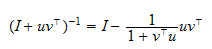Proof

Let us first prove the "if" part. The assumptionensures that the ratioand the proposed inverseare well-defined. Moreover, the latter satisfies the definition of inverse (a matrix multiplied by its inverse gives the identity matrix):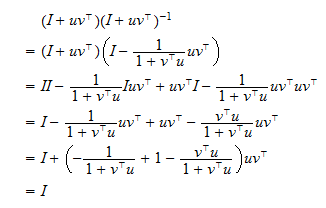Let us now prove the "only if" part. SupposeorThe latter equality implies. Post-multiply the matrixby:Thus, the linear combination of the columns ofwith coefficients taken from the non-zero vectorgives the zero vector. As a consequence, the columns ofare not linearly independent, the matrix is not full-rank, hence not invertible.

Note the dimensions of the matrix products involved in this inversion lemma:

• the rank one update, that is, the productis;

• the invertibility condition is based on the productwhose dimension is; in other words, it is a scalar.

## Sherman-Morrison formula

The Sherman-Morrison formula, shown in the next proposition, generalizes the previous inversion lemma by considering rank one updates to a generic invertible matrix(instead of only considering rank one updates to the identity matrix).

Proposition Letbe ainvertible matrix andandtwocolumn vectors. The rank one updateis invertible if and only if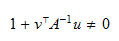When it is invertible, its inverse is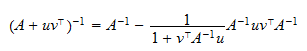Proof

Note that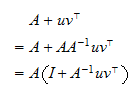In other words, we can write a rank one update toas the product ofand a rank one update to the identity matrix (the update is performed with the column vectorsand). Thus, by the standard result on the inverse of a product, we have thatis invertible if and only if the two factors of the product, that is,andare invertible. Butis invertible by assumption, and the condition for the invertibility of a rank one update to the identity matrix has been derived in the previous proposition: the rank one update is invertible if and only ifWhen it is invertible, its inverse is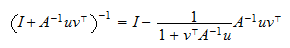As a consequence,The Sherman-Morrison formula is very useful not only because rank one updates are found in many theorems in matrix algebra, but also because it can be a computationally efficient way of calculating the inversewhenhas already been calculated. The number of arithmetic operations needed to computefrom scratch is proportional to, while the number of operations needed to update the inverse with Sherman-Morrison formula is proportional to. Even if in the latter case the constant of proportionality is higher than in the former, whenis sufficiently large, the computational cost of the update is much lower.

## Woodbury matrix identity

Another useful matrix inversion lemma goes under the name of Woodbury matrix identity, which is presented in the following proposition.

Proposition Letbe ainvertible matrix,andtwomatrices, andaninvertible matrix. Ifis invertible, thenis invertible and its inverse isProof

The condition thatexists ensures that the proposed inverse is well-defined. We need to check that the proposed inverse satisfies the definition of inverse (a matrix multiplied by its inverse gives the identity matrix):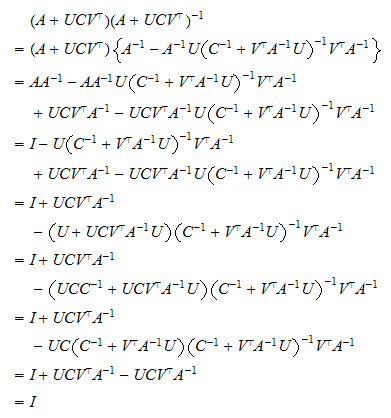Note that whenand, the Woodbury matrix identity coincides with the Sherman Morrison formula. Therefore, the latter is a special case of the former.

The reasons why this inversion lemma is worth knowing are similar to those we have explained for the Sherman Morrison formula: it is often used in matrix algebra, and it saves computations whenis already known (andis significantly smaller than).